# Movement sensing using accelerometer

You may not have heard of accelerometers, but you must experience them in everyday life. For example, when you rotate your mobile phone, you may find the screen display rotate from portrait to landscape. And it's due to the accelerometer inside the phone. It senses the movement and then responds to it accordingly. Now let’s take a closer look at it.

Learning goals

• Understand how an accelerometer senses movements.

## 🔸New component​

### Accelerometer​

The accelerometer, aka 3-axis accelerometer, measures motion and gives the accelerations on x, y, z-axis. You could infer the movement according to the values.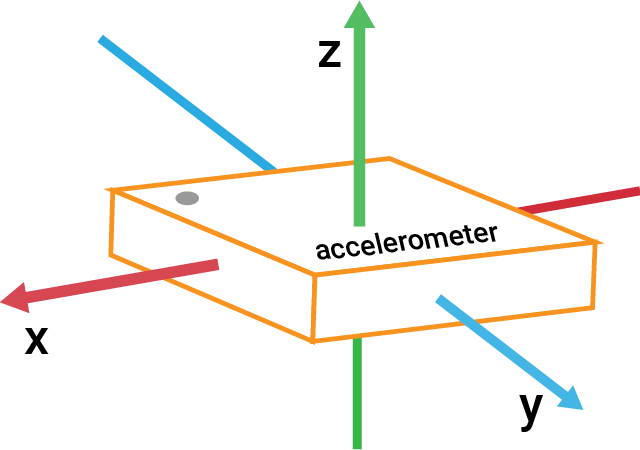It can sense gravity and measure motion, like vibration, rotation. The sensor contains a structure whose part moves when an acceleration is applied. It will change the internal capacitance of the sensor, and thus voltage in circuits also changes.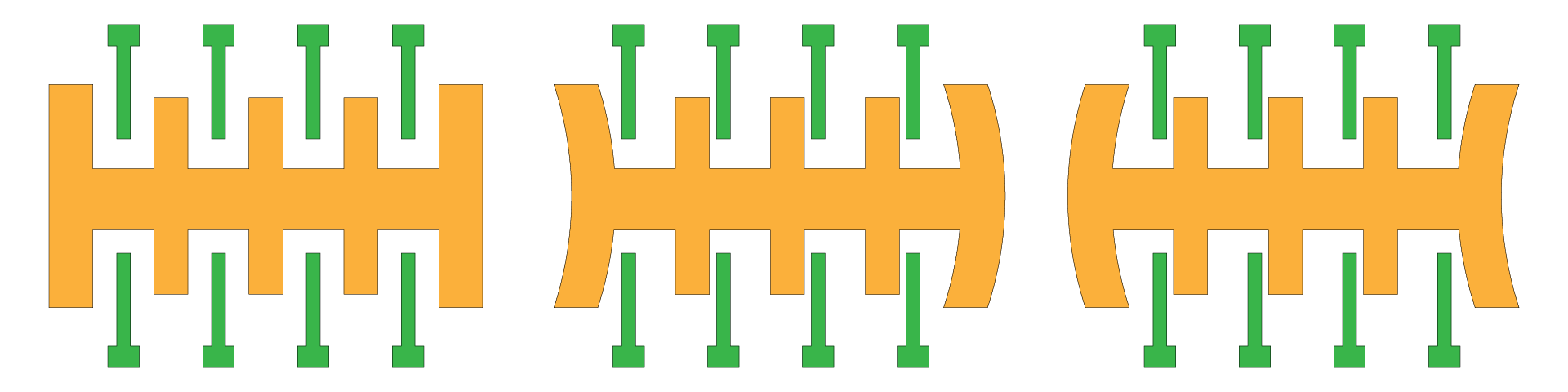To better understand how the accelerometer works, let’s imagine a small ball inside a vacuum box. There are several situations:

• There is no gravity in a vacuum box, so the ball floats in the middle.
• If you suddenly move the box to the left, the ball will move towards the opposite direction and hit the box. This is similar to the experience when you take a bus. If the bus suddenly speeds up, your body feels a force backward and then moves backward. This force is known as inertial force.
• If air comes into the box, the ball will fall due to gravity.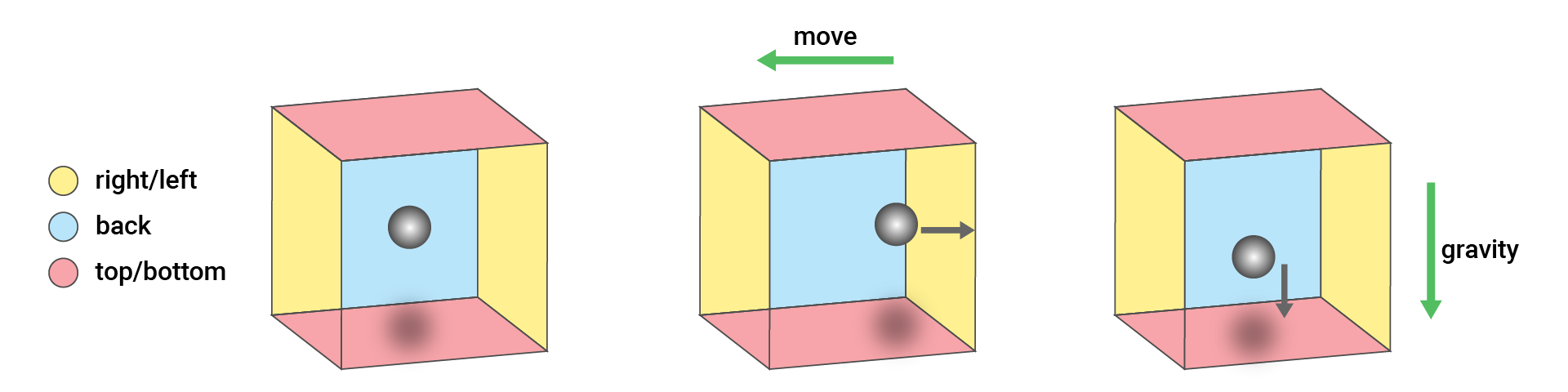Now let’s go back to the sensor.
• If the sensor is still, it only feels the gravity. When the sensor faces up on the table, it gets a force downwards on the z-axis. When the sensor is perpendicular to the table, there will be a force on the x or y-axis.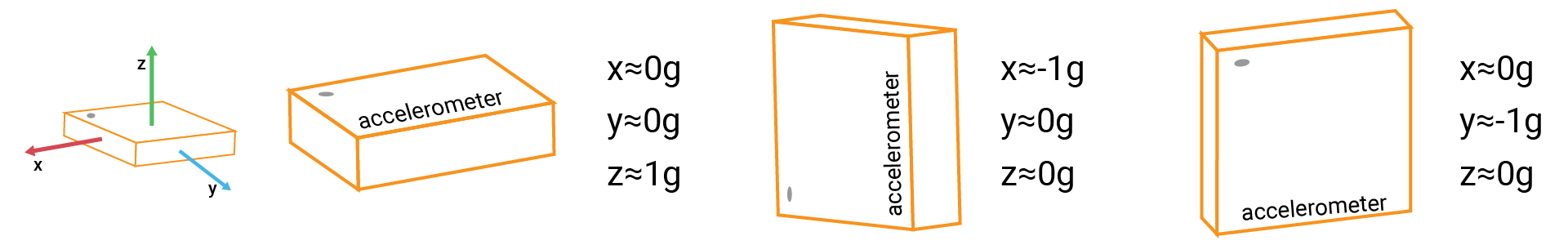• If the sensor moves toward some directions, the sensor should sense corresponding inertial force and gravity force.

The acceleration describes the change of velocity with time, in meters per second squared (m/s2). It has directions and can be either positive or negative. The gravitational acceleration on earth equals 1g (9.8m/s2). The accelerations measured by the sensor are in g.

The accelerometer LIS3DH supports I2C and SPI communication, but the module on your kit can only connects to I2C interface. The data it sends over the bus is raw values ranging from -32768 to 32767 (16-bit). Then the values will be converted to the acceleration value proportionally according to the measurement range.

The sensor provides several measurement ranges: ±2g, ±4g, ±8g, ±16g. If you choose ±2g (which is the default range), -32768 equals -2g and 32768 equals 2g, which gives more precise readings within the limited range.

## 🔸Circuit - accelerometer module​

The accelerometer module is connected to I2C0 (SCL0 and SDA0).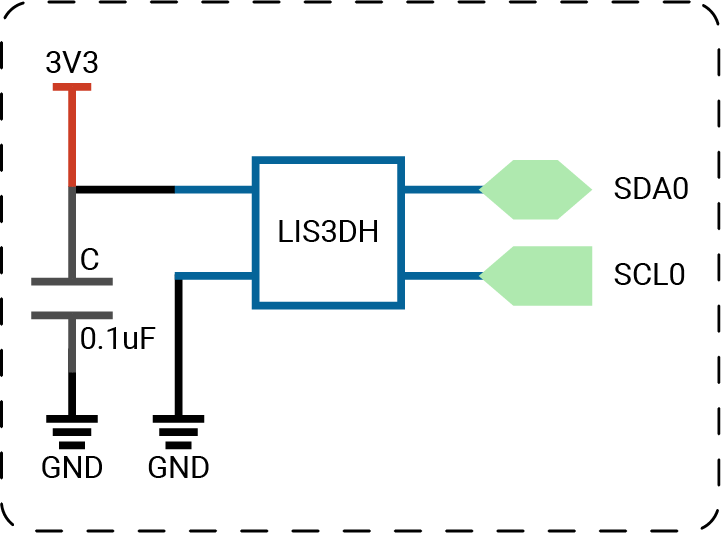##### note

The circuits above are simplified versions for your reference.

## 🔸Preparation​

Class

`LIS3DH` - it is the library for LIS3DH accelerometer. It provides predefined methods for you to get readings from the sensor in an easy way.

MethodExplanation
`init(_:address:)`Initialize the sensor to start the communication.
Parameters:
- `id`: I2C interface that the sensor connects to.
- `address`: the sensor's address. 0x18 by default.
`readXYZ()`Read the acceleration on 3-axis.
Return value:
Accelerations on x, y, z-axis on float.

## 🔸Projects​

Let’s use the sensor to sense movements. As you move or tilt your board in different directions, the values printed on the window change accordingly. You can have a better idea of how the accelerations change with movement.

Example code

``// Import SwiftIO to set the communication and MadBoard to use pin id. import SwiftIOimport MadBoard// Import LIS3DH to read the accelerations from the sensor.import LIS3DH// Initialize the I2C pins and the sensor.let i2c = I2C(Id.I2C0)let acc = LIS3DH(i2c)// Read the accelerations and each of them.while true {    let value = acc.readXYZ()    print("x: \(value.x)g")    print("y: \(value.y)g")    print("z: \(value.z)g")    print("\n")    sleep(ms: 1000)}``

### 2. Digital dice​

Let's build a dice with the accelerometer. You can shake or tilt the sensor to change the accelerations. It simulates the process of rolling a dice. After that, you put it back on your table or keep it in a horizontal position, you should get a random number from 1 to 6.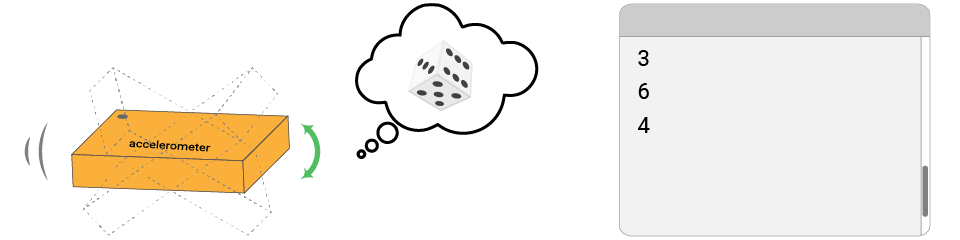Example code

``// Import SwiftIO to set input and output.import SwiftIO// Import MadBoard to use the id of the pins.import MadBoard// Import this driver to read accelerations on x, y, z-axis.import LIS3DH// Initialize an I2C interface and use it to set the sensor.let i2c = I2C(Id.I2C0)let dice = LIS3DH(i2c)// Initialize an LED used as an indicator when shaking the sensor.let indicator = DigitalOut(Id.D19)// Create a variable to store the time after the sensor stops movement.var steadyCount = 999// Read accelerations to judge if the sensor is in motion. // Once the movement stops, a random number prints out.while true {    let diceValue = dice.readXYZ()        if abs(diceValue.x) > 0.3 || abs(diceValue.y) > 0.3 {        indicator.high()        steadyCount = 0    } else {        steadyCount += 1        if steadyCount == 50 {            indicator.low()            print(Int.random(in: 1...6))        }    }    sleep(ms: 5)}``

Code analysis

``let indicator = DigitalOut(Id.D19)``

This LED is served as an indicator when shaking the sensor.

``var steadyCount = 999``

This statement declares a new variable used to store the time. While you shake the sensor, the accelerations change in undetermined states. This count can infer if the sensor comes to a horizontal position after a shake.

``if abs(diceValue.x) > 0.3 || abs(diceValue.y) > 0.3 {    indicator.high()    steadyCount = 0} else {    steadyCount += 1    if steadyCount == 50 {        indicator.low()        print(Int.random(in: 1...6))    }}``

An if-else statement is used to judge the sensor's state.

• The accelerations should increase in magnitude when you shake the sensor. Since the accelerations have directions, you need to use `abs` to get absolute values. During that time, you turn on the LED as an indicator. Besides, the variable `steadyCount` is set to 0 and always be 0 when the sensor is still in motion.

• Once you stop shaking the sensor, the accelerations on the x and y-axis decrease and should be lower than the threshold. Then the count starts to increase until it exceeds the set value. If so, it means the sensor is kept in a horizontal position. This is similar to the period after a dice is thrown. At last, you should see a number printed on your serial window.

In this exercise, the key point is to decide when the sensor is no longer in motion. Here you store the time to make sure the state lasts for a certain period.